# Ohlson’s O-Score

Ohlson’s O-Score is a default predition model similar to the more well-known Altman z-score. The Ohlson O-score model was introduced by James Ohlson in 1980 in an article in the Journal of Accounting research. The objective of the O-score is to predict whether or not a company is likely to go bankrupt in the near future. As such, the Ohlson bankruptcy model measures distress risk. To do this kind of prediction, the Ohlson model only uses a company’s financial ratios, which are publicly available.

On this page, we discuss the formula of the Ohlson model and provide a numerical example in Exce that implements the Ohlson bankruptcy prediction model. The Excel template is available for download at the bottom of the page.

## Ohlson model definition

The Ohlson O-score is based on a linear factor model. In particular, using 9 different business ratios that can be obtained from companies’ financial statements, one can calculate the O-score. Interestingly, two of the factor (the dummy factors) in the 9-factor model are not really necessary, as their impact on the formula is typically zero.

The formula for Ohlson’s O-score is the following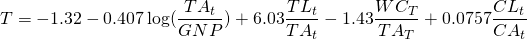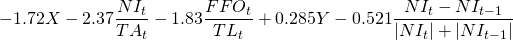where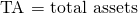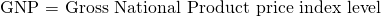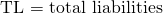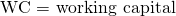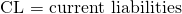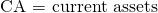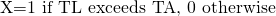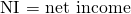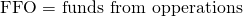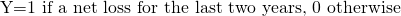All the above data can be retrieved from companies’ financial statements, except for the GNP price level index. Ohlson’s model uses this particular variable to adjust total assets for changes in the price index level (i.e. inflation).

## Ohlson O-score probability of default

To convert the O-score into a probability of default, we need to do the following: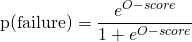Although the above formula looks really complicated, it is very easy to implement the analysis in Excel. At the bottom of the page, we illustrate how a simple spreadsheet can be used to implement the Ohlson O-score calculation.

## Summary

We presented the formula for Ohlson’s O-score, a widely used model to predict distress risk among publicly traded companies. While the formula looks very complex, the model can easily be implemented in Excel.

### Ohlson O-score calculation

Want to have an implementation in Excel? Download the Excel file: Ohlson O-score template